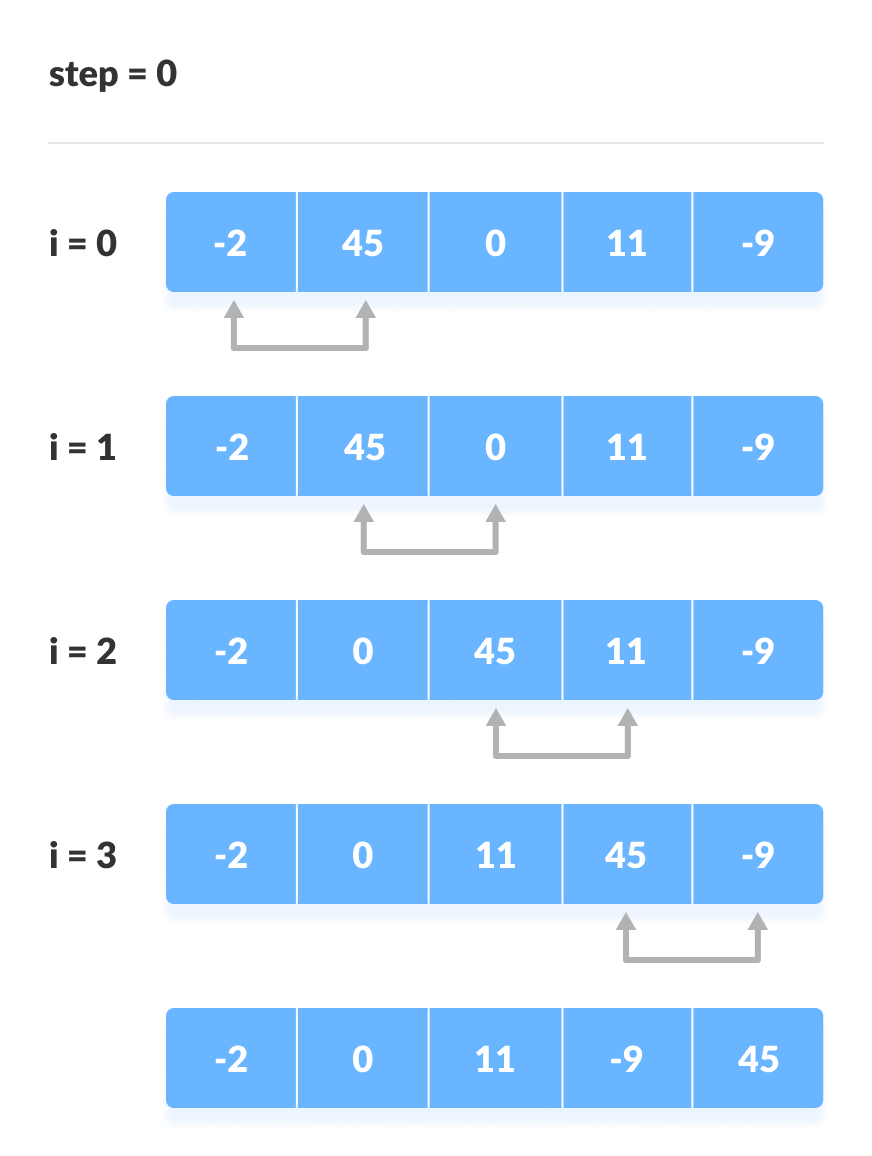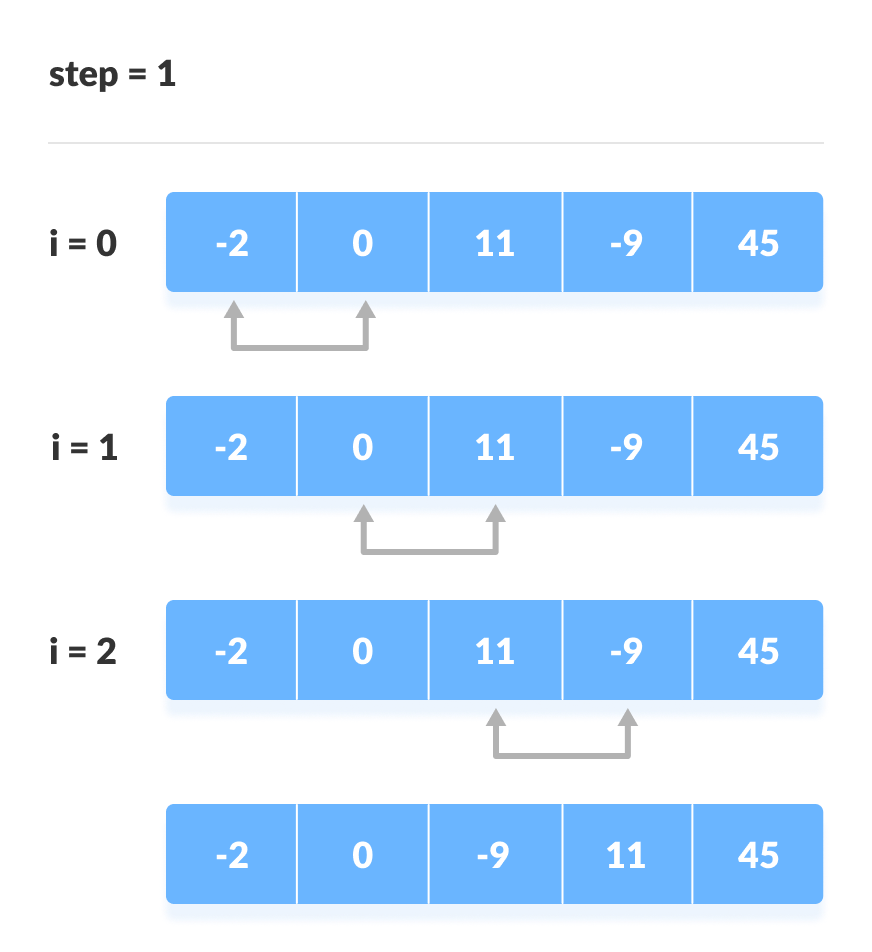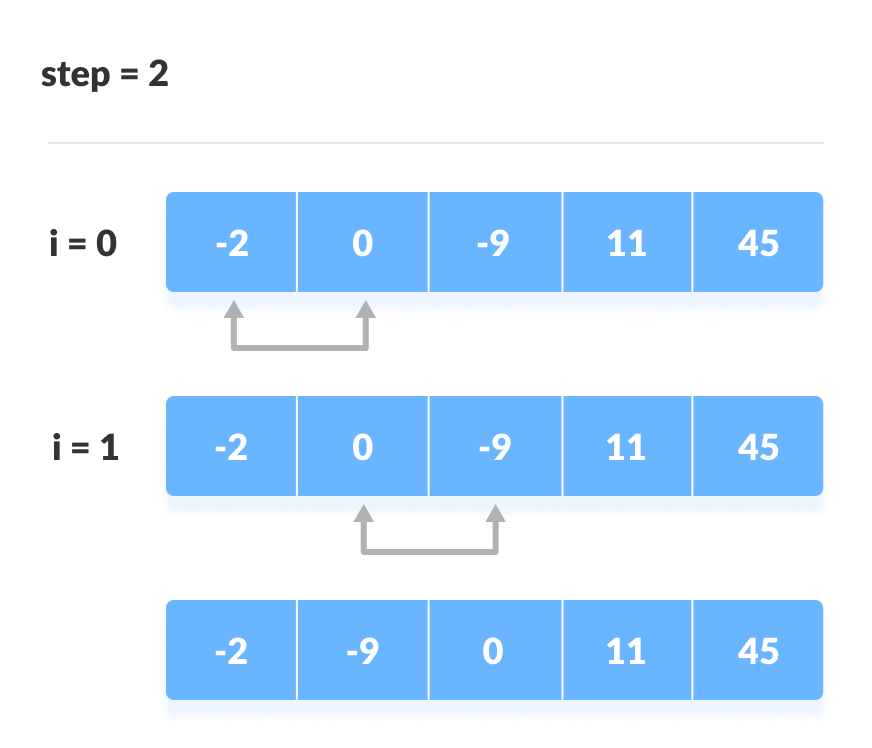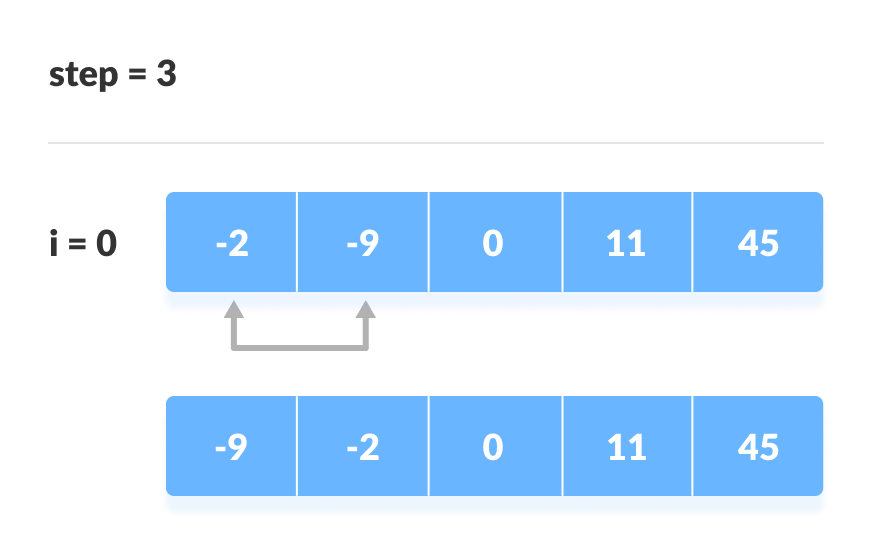# Bubble Sort Algorithm

In this tutorial, you will learn how bubble sort works. Also, you will find working examples of bubble sort in C, C++, Java and Python.

Bubble sort is an algorithm that compares the adjacent elements and swaps their positions if they are not in the intended order. The order can be ascending or descending.

## How Bubble Sort Works?

1. Starting from the first index, compare the first and the second elements.If the first element is greater than the second element, they are swapped.

Now, compare the second and the third elements. Swap them if they are not in order.

The above process goes on until the last element.2. The same process goes on for the remaining iterations. After each iteration, the largest element among the unsorted elements is placed at the end.

In each iteration, the comparison takes place up to the last unsorted element.

The array is sorted when all the unsorted elements are placed at their correct positions.## Bubble Sort Algorithm

``````bubbleSort(array)
for i <- 1 to indexOfLastUnsortedElement-1
if leftElement > rightElement
swap leftElement and rightElement
end bubbleSort``````

## Python, Java and C/C++ Examples

``````# Bubble sort in Python

def bubbleSort(array):
for i in range(len(array)):
for j in range(0, len(array)-i-1):
# To sort in descending order, change > to < in this line.
if array[j] > array[j+1]:
array[j], array[j+1] = array[j+1], array[j]

data = [-2, 45, 0, 11, -9]
bubbleSort(data)
print("Sorted Array in Ascending Order:")
print(data)``````
``````// Bubble sort in Java

class BubbleSort{
void bubbleSort(int array[]){
int size = array.length;
for (int i = 0; i < size-1; i++)
for (int j = 0; j < size-i-1; j++)
// To sort in descending order, change > to < in this line.
if (array[j] > array[j+1]){
int temp = array[j];
array[j] = array[j+1];
array[j+1] = temp;
}
}

public static void main(String args[]){
int[] data = {-2, 45, 0, 11, -9};
BubbleSort bs = new BubbleSort();
bs.bubbleSort(data);
System.out.println("Sorted Array in Ascending Order:");
System.out.println(Arrays.toString(data));
}
}
``````
``````// Bubble sort in C

#include <stdio.h>

void bubbleSort(int array[], int size){
for(int step=0; step<size-1; ++step){
for(int i=0; i<size-step-1; ++i){
// To sort in descending order, change">" to "<".
if(array[i]>array[i+1]){
int temp=array[i];
array[i]=array[i+1];
array[i+1]=temp;
}
}
}
}
void printArray(int array[], int size){
for(int i=0; i<size; ++i){
printf("%d  ", array[i]);
}
printf("\n");
}
int main(){
int data[] = {-2, 45, 0, 11, -9};
int size = sizeof(data)/sizeof(data);
bubbleSort(data, size);
printf("Sorted Array in Ascending Order:\n");
printArray(data, size);
}``````
``````// Bubble sort in C++

#include <iostream>
using namespace std;

void bubbleSort(int array[], int size){
for(int step=0; step<size-1; ++step){
for(int i=0; i<size-step-1; ++i){
// To sort in descending order, change > to < in this line.
if(array[i]>array[i+1]){
int temp=array[i];
array[i]=array[i+1];
array[i+1]=temp;
}
}
}
}
void printArray(int array[], int size){
for(int i=0; i<size; ++i){
cout<<"  "<<array[i];
}
cout<<"\n";
}
int main(){
int data[] = {-2, 45, 0, 11, -9};
int size = sizeof(data)/sizeof(data);
bubbleSort(data, size);
cout<<"Sorted Array in Ascending Order:\n";
printArray(data, size);
}``````

## Optimized Bubble Sort

In the above code, all possible comparisons are made even if the array is already sorted. It increases the execution time.

The code can be optimized by introducing an extra variable swapped. After each iteration, if there is no swapping taking place then, there is no need for performing further loops.

In such case, variable swapped is set false. Thus, we can prevent further iterations.

Algorithm for optimized bubble sort is

``````bubbleSort(array)
swapped <- false
for i <- 1 to indexOfLastUnsortedElement-1
if leftElement > rightElement
swap leftElement and rightElement
swapped <- true
end bubbleSort
``````

### Optimized Bubble Sort Examples

``````# Optimized bubble sort in Python

def bubbleSort(array):
for i in range(len(array)):
swapped = True
for j in range(0, len(array)-i-1):
# To sort in descending order, change > to < in this line.
if array[j] > array[j+1]:
array[j], array[j+1] = array[j+1], array[j]
swapped = False
if swapped == True:
break

data = [-2, 45, 0, 11, -9]
bubbleSort(data)
print("Sorted Array in Ascending Order:")
print(data)
``````
``````// Optimized bubble sort in Java

import java.util.Scanner;

class BubbleSort {
void bubbleSort(int array[]) {
int size = array.length;
for (int i = 0; i < size - 1; i++) {
boolean swapped = true;
for (int j = 0; j < size - i - 1; j++) {
// To sort in descending order, change > to < in this line.
if (array[j] > array[j + 1]) {
int temp = array[j];
array[j] = array[j + 1];
array[j + 1] = temp;
swapped = false;
}
}
if (swapped == true)
break;
}
}

public static void main(String args[]) {
int[] data = { -2, 45, 0, 11, -9 };
BubbleSort bs = new BubbleSort();
bs.bubbleSort(data);
System.out.println("Sorted Array in Ascending Order:");
System.out.println(Arrays.toString(data));
}
}
``````
``````// Optimized bubble sort in C

#include <stdio.h>

void bubbleSort(int arrayay[], int size)
{
for (int step = 0; step & lt; size - 1; ++step)
{
// The variable "swapped" is introduced for optimization.
int swapped = 0;
for (int i = 0; i & lt; size - step - 1; ++i)
{
// To sort in descending order, change > to < in this line.
if (arrayay[i] & gt; arrayay[i + 1])
{
int temp = arrayay[i];
arrayay[i] = arrayay[i + 1];
arrayay[i + 1] = temp;
swapped = 1;
}
}
// If there is not swapping in the last swap, then the array is already sorted.
if (swapped == 0)
break;
}
}
void printarrayay(int arrayay[], int size)
{
for (int i = 0; i & lt; size; ++i)
{
printf("%d  ", arrayay[i]);
}
printf("\n");
}
int main()
{
int data[] = {-2, 45, 0, 11, -9};
int size = sizeof(data) / sizeof(data);
bubbleSort(data, size);
printf("Sorted Array in Ascending Order:\n");
printarrayay(data, size);
}``````
``````// Optimized bubble sort in C++

#include <iostream>
using namespace std;

void bubbleSort(int array[], int size)
{
for (int step = 0; step < size - 1; ++step)
{
int swapped = 0;
for (int i = 0; i < size - step - 1; ++i)
{
// To sort in descending order, change > to < in this line.
if (array[i] > array[i + 1])
{
int temp = array[i];
array[i] = array[i + 1];
array[i + 1] = temp;
swapped = 1;
}
}
// If there is not swapping in the last swap, then the array is already sorted.
if (swapped == 0)
break;
}
}
void printArray(int array[], int size)
{
for (int i = 0; i < size; ++i)
{
cout << "  " << array[i];
}
cout << "\n";
}
int main()
{
int data[] = {-2, 45, 0, 11, -9};
int size = sizeof(data) / sizeof(data);
bubbleSort(data, size);
cout << "Sorted Array in Ascending Order:\n";
printArray(data, size);
}``````

## Complexity

Bubble Sort is one of the simplest sorting algorithms. Two loops are implemented in the algorithm.

Cycle Number of Comparisons
1st (n-1)
2nd (n-2)
3rd (n-3)
....... ......
last 1

Number of comparisons:`(n-1) + (n-2) + (n-3) +.....+ 1 = n(n-1)/2` nearly equals to `n2`

Complexity: `O(n2)`

Also, we can analyze the complexity by simply observing the number of loops. There are 2 loops so the complexity is `n*n = n2`

Time Complexities:

• Worst Case Complexity: `O(n2)`

If we want to sort in ascending order and the array is in descending order then, the worst case occurs.

• Best Case Complexity: `O(n)`

If the array is already sorted, then there is no need for sorting.

• Average Case Complexity: `O(n2)`

It occurs when the elements of the array are in jumbled order (neither ascending nor descending).

Space Complexity:

Space complexity is `O(1)` because an extra variable temp is used for swapping.

In the optimized algorithm, the variable swapped adds to the space complexity thus, making it `O(2)`.

## Bubble Sort Applications

Bubble sort is used in the following cases where

1. the complexity of the code does not matter.
2. a short code is preferred.